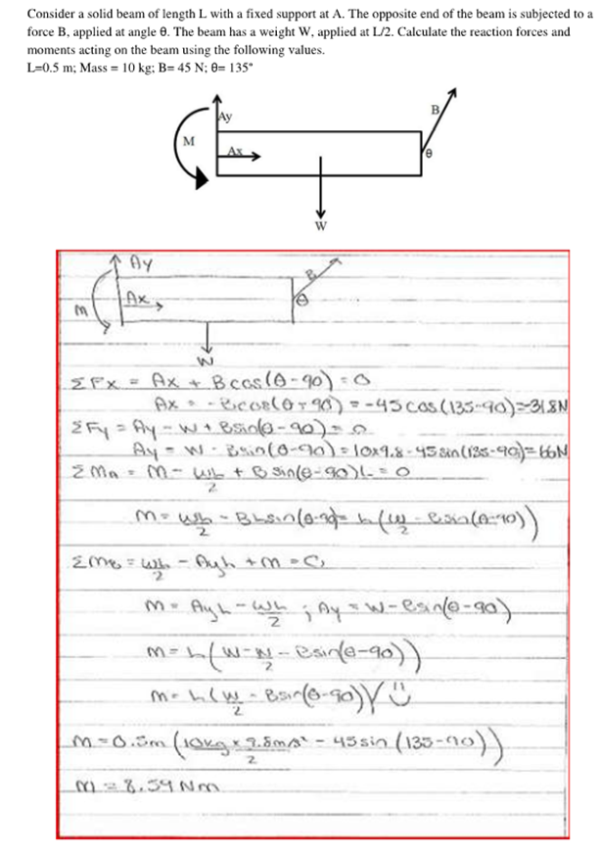Consider a solid beam of length L with a fixed support at A. The opposite end of the beam is subected to a force B, applied at angle theta. The beam has a weight W, applied at L/2. Calculate the reaction forrces and moments acting on the beam using the following values, L=0.5 m, Mass=10 kg, B=45 N, theta=135 degrees.Consider a solid beam of length L with a fixed support at A. The opposite end of the beam is subected to a force B, applied at angle theta. The beam has a weight W, applied at L/2. Calculate the reaction forrces and moments acting on the beam using the following values, L=0.5 m, Mass=10 kg, B=45 N, theta=135 degrees.

biomechanics page 1 biomechanics biomechanics biomechanics biomechanics biomechanics biomechanics biomechanics biomechanics page 2